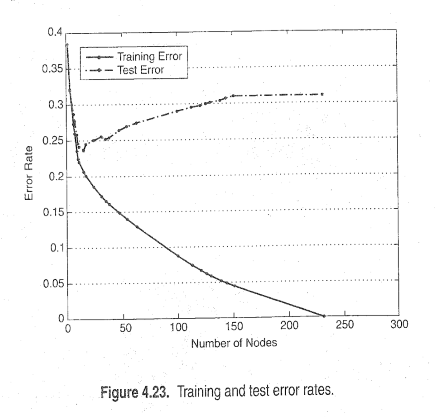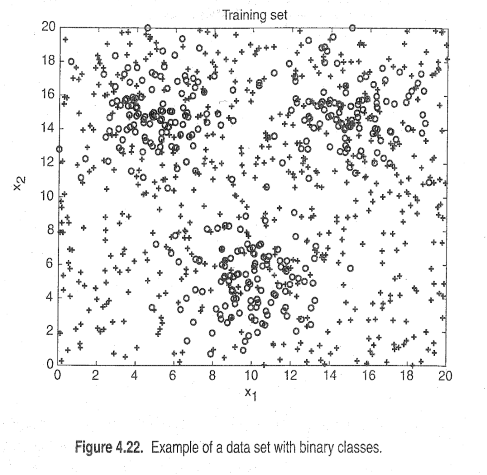# Decision Tree Validation

Member Posts: 4Contributor I
edited November 2018 in Help

Hello experts!

I'm a newby in rapidminer . Can anyone help me how to illustrate  the following diagram with rapidminer???I generated 3000 datapoints with python.my datapoins belong to two different classes. class one is generated from a mixture of three gaussian distributions and class two is generated from a uniform distribution. 1200 datapoins for class one and 1800 datapoins for class two. 30% of datapoints are chosen for training,while the remaining  70% are used for testing. I used  a decision tree classifier .I'm sorry if this is not the correct place for post this doubt, but like I said before, I'm new here.

Thanks for the attention!

Tagged:

• Moderator, RapidMiner Certified Analyst, RapidMiner Certified Expert, Member Posts: 1,635Unicorn

You would use an "optimize parameters" operator that iterates over the number of tree nodes. Then use the "log" operator to preserve the performance measure and the number of nodes.  In theory, if you do this with a "split data" operator and observe the outcome on a single model training set (with no cross validation) then you'll observe that your error rate only decreases as you add more nodes. Then run it again on your test set inside a cross-validation and you will see that error decreases at first given more nodes but then increases at some point once you get into the complexity region of overfit trees.  The specific results will depend on your data and won't look exactly like the attached graphic but the same general pattern should be true.

Brian T.
Lindon Ventures
Data Science Consulting from Certified RapidMiner Experts
• Member Posts: 4Contributor I
• Member Posts: 4Contributor Iwhere is wrong???

• Moderator, RapidMiner Certified Analyst, RapidMiner Certified Expert, Member Posts: 1,635Unicorn

If you can post the process itself rather than the screenshots it is easier to troubleshoot.  You can go to the file menu and then choose "export process" and it will output a file called something.rmp which is a small file containing the full process.

Brian T.
Lindon Ventures
Data Science Consulting from Certified RapidMiner Experts
• Member Posts: 4Contributor I
`<?xml version="1.0" encoding="UTF-8"?><process version="7.3.000">  <context>    <input/>    <output/>    <macros/>  </context>  <operator activated="true" class="process" compatibility="7.3.000" expanded="true" name="Process">    <parameter key="logverbosity" value="init"/>    <parameter key="random_seed" value="2001"/>    <parameter key="send_mail" value="never"/>    <parameter key="notification_email" value=""/>    <parameter key="process_duration_for_mail" value="30"/>    <parameter key="encoding" value="SYSTEM"/>    <process expanded="true">      <operator activated="true" class="advanced_file_connectors:read_arff" compatibility="7.3.000" expanded="true" height="68" name="Read ARFF" width="90" x="45" y="187">        <parameter key="data_file" value="C:\Users\fatemeh yaghoubi\Desktop\term one\poroject_datamining\TREE\tree eleven\rapidminer.arff"/>        <parameter key="encoding" value="SYSTEM"/>        <parameter key="read_not_matching_values_as_missings" value="true"/>        <list key="data_set_meta_data_information"/>        <parameter key="attribute_names_already_defined" value="false"/>        <parameter key="decimal_character" value="."/>        <parameter key="grouped_digits" value="false"/>        <parameter key="grouping_character" value=","/>      </operator>      <operator activated="true" class="set_role" compatibility="7.3.000" expanded="true" height="82" name="Set Role" width="90" x="246" y="187">        <parameter key="attribute_name" value="class"/>        <parameter key="target_role" value="label"/>        <list key="set_additional_roles">          <parameter key="class" value="label"/>        </list>      </operator>      <operator activated="true" class="loop" compatibility="7.3.000" expanded="true" height="124" name="Loop" width="90" x="447" y="187">        <parameter key="set_iteration_macro" value="false"/>        <parameter key="macro_name" value="iteration"/>        <parameter key="macro_start_value" value="1"/>        <parameter key="iterations" value="600"/>        <parameter key="limit_time" value="false"/>        <parameter key="timeout" value="1"/>        <process expanded="true">          <operator activated="true" class="optimize_parameters_grid" compatibility="7.3.000" expanded="true" height="145" name="Optimize Parameters (Grid)" width="90" x="380" y="136">            <list key="parameters">              <parameter key="Performance.classification_error" value="true,false"/>              <parameter key="Decision Tree.minimal_leaf_size" value="[1.0;100.0;10;linear]"/>            </list>            <parameter key="error_handling" value="fail on error"/>            <process expanded="true">              <operator activated="true" class="split_validation" compatibility="7.3.000" expanded="true" height="124" name="Validation" width="90" x="313" y="85">                <parameter key="create_complete_model" value="false"/>                <parameter key="split" value="relative"/>                <parameter key="split_ratio" value="0.7"/>                <parameter key="training_set_size" value="100"/>                <parameter key="test_set_size" value="-1"/>                <parameter key="sampling_type" value="shuffled sampling"/>                <parameter key="use_local_random_seed" value="false"/>                <parameter key="local_random_seed" value="1992"/>                <process expanded="true">                  <operator activated="true" class="parallel_decision_tree" compatibility="7.3.000" expanded="true" height="82" name="Decision Tree" width="90" x="112" y="136">                    <parameter key="criterion" value="gain_ratio"/>                    <parameter key="maximal_depth" value="20"/>                    <parameter key="apply_pruning" value="true"/>                    <parameter key="confidence" value="0.25"/>                    <parameter key="apply_prepruning" value="true"/>                    <parameter key="minimal_gain" value="Infinity"/>                    <parameter key="minimal_leaf_size" value="100"/>                    <parameter key="minimal_size_for_split" value="4"/>                    <parameter key="number_of_prepruning_alternatives" value="3"/>                  </operator>                  <connect from_port="training" to_op="Decision Tree" to_port="training set"/>                  <connect from_op="Decision Tree" from_port="model" to_port="model"/>                  <portSpacing port="source_training" spacing="0"/>                  <portSpacing port="sink_model" spacing="0"/>                  <portSpacing port="sink_through 1" spacing="0"/>                </process>                <process expanded="true">                  <operator activated="true" class="apply_model" compatibility="7.3.000" expanded="true" height="82" name="Apply Model" width="90" x="45" y="136">                    <list key="application_parameters"/>                    <parameter key="create_view" value="false"/>                  </operator>                  <operator activated="true" class="performance_classification" compatibility="7.3.000" expanded="true" height="82" name="Performance" width="90" x="222" y="136">                    <parameter key="main_criterion" value="classification_error"/>                    <parameter key="accuracy" value="false"/>                    <parameter key="classification_error" value="false"/>                    <parameter key="kappa" value="false"/>                    <parameter key="weighted_mean_recall" value="false"/>                    <parameter key="weighted_mean_precision" value="false"/>                    <parameter key="spearman_rho" value="false"/>                    <parameter key="kendall_tau" value="false"/>                    <parameter key="absolute_error" value="false"/>                    <parameter key="relative_error" value="false"/>                    <parameter key="relative_error_lenient" value="false"/>                    <parameter key="relative_error_strict" value="false"/>                    <parameter key="normalized_absolute_error" value="false"/>                    <parameter key="root_mean_squared_error" value="false"/>                    <parameter key="root_relative_squared_error" value="false"/>                    <parameter key="squared_error" value="false"/>                    <parameter key="correlation" value="false"/>                    <parameter key="squared_correlation" value="false"/>                    <parameter key="cross-entropy" value="false"/>                    <parameter key="margin" value="false"/>                    <parameter key="soft_margin_loss" value="false"/>                    <parameter key="logistic_loss" value="false"/>                    <parameter key="skip_undefined_labels" value="true"/>                    <parameter key="use_example_weights" value="true"/>                    <list key="class_weights"/>                  </operator>                  <connect from_port="model" to_op="Apply Model" to_port="model"/>                  <connect from_port="test set" to_op="Apply Model" to_port="unlabelled data"/>                  <connect from_op="Apply Model" from_port="labelled data" to_op="Performance" to_port="labelled data"/>                  <connect from_op="Performance" from_port="performance" to_port="averagable 1"/>                  <portSpacing port="source_model" spacing="0"/>                  <portSpacing port="source_test set" spacing="0"/>                  <portSpacing port="source_through 1" spacing="0"/>                  <portSpacing port="sink_averagable 1" spacing="0"/>                  <portSpacing port="sink_averagable 2" spacing="0"/>                </process>              </operator>              <connect from_port="input 1" to_op="Validation" to_port="training"/>              <connect from_op="Validation" from_port="model" to_port="result 2"/>              <connect from_op="Validation" from_port="training" to_port="result 1"/>              <connect from_op="Validation" from_port="averagable 1" to_port="performance"/>              <portSpacing port="source_input 1" spacing="0"/>              <portSpacing port="source_input 2" spacing="0"/>              <portSpacing port="sink_performance" spacing="0"/>              <portSpacing port="sink_result 1" spacing="0"/>              <portSpacing port="sink_result 2" spacing="0"/>              <portSpacing port="sink_result 3" spacing="0"/>            </process>          </operator>          <connect from_port="input 1" to_op="Optimize Parameters (Grid)" to_port="input 1"/>          <connect from_op="Optimize Parameters (Grid)" from_port="performance" to_port="output 1"/>          <connect from_op="Optimize Parameters (Grid)" from_port="parameter" to_port="output 2"/>          <connect from_op="Optimize Parameters (Grid)" from_port="result 1" to_port="output 3"/>          <portSpacing port="source_input 1" spacing="0"/>          <portSpacing port="source_input 2" spacing="0"/>          <portSpacing port="sink_output 1" spacing="0"/>          <portSpacing port="sink_output 2" spacing="0"/>          <portSpacing port="sink_output 3" spacing="0"/>          <portSpacing port="sink_output 4" spacing="0"/>        </process>      </operator>      <operator activated="true" class="log" compatibility="7.3.000" expanded="true" height="82" name="Log" width="90" x="581" y="187">        <parameter key="filename" value="Mylog"/>        <list key="log">          <parameter key="Number Of Nodes" value="operator.Decision Tree.parameter.minimal_leaf_size"/>          <parameter key="Error Rate" value="operator.Performance.parameter.classification_error"/>        </list>        <parameter key="sorting_type" value="none"/>        <parameter key="sorting_k" value="100"/>        <parameter key="persistent" value="false"/>      </operator>      <connect from_op="Read ARFF" from_port="output" to_op="Set Role" to_port="example set input"/>      <connect from_op="Set Role" from_port="example set output" to_op="Loop" to_port="input 1"/>      <connect from_op="Loop" from_port="output 1" to_op="Log" to_port="through 1"/>      <connect from_op="Log" from_port="through 1" to_port="result 1"/>      <portSpacing port="source_input 1" spacing="0"/>      <portSpacing port="sink_result 1" spacing="0"/>      <portSpacing port="sink_result 2" spacing="0"/>    </process>  </operator></process>`
• Moderator, RapidMiner Certified Analyst, RapidMiner Certified Expert, Member Posts: 1,635Unicorn

I don't have access to your data, so I modified the process using the sample Titanic dataset.  This dataset is too small to see much of a difference in the tree performance here, but the setup of this process is now correct.  If you do this on a larger dataset you should see more of a divergence between training vs testing error as shown in the original graphic.  The training error only goes down.  The testing error goes down but then goes back up once overfitting occurs.

Brian T.
Lindon Ventures
Data Science Consulting from Certified RapidMiner Experts